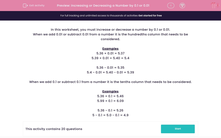# Increasing or Decreasing a Number by 0.1 or 0.01

In this worksheet, students practise increasing or decreasing the given numbers by 0.1 or 0.01.This content is premium and exclusive to EdPlace subscribers.Key stage:  KS 2

Curriculum topic:   Maths and Numerical Reasoning

Curriculum subtopic:   Mixed Problems

Difficulty level:#### Worksheet Overview

In this worksheet, you must increase or decrease a number by 0.1 or 0.01.

When we add 0.01 or subtract 0.01 from a number it is the hundredths column that needs to be considered.

Examples

5.36 + 0.01 = 5.37

5.39 + 0.01 = 5.40 = 5.4

5.36 - 0.01 = 5.35

5.4 - 0.01 = 5.40 - 0.01 = 5.39

When we add 0.1 or subtract 0.1 from a number it is the tenths column that needs to be considered.

Examples

5.36 + 0.1 = 5.46

5.99 + 0.1 = 6.09

5.36 - 0.1 = 5.26

5 - 0.1 = 5.0 - 0.1 = 4.9

### What is EdPlace?

We're your National Curriculum aligned online education content provider helping each child succeed in English, maths and science from year 1 to GCSE. With an EdPlace account you’ll be able to track and measure progress, helping each child achieve their best. We build confidence and attainment by personalising each child’s learning at a level that suits them.

Get started